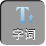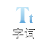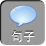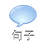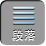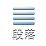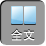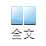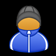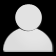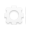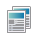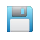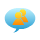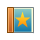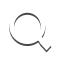-AA+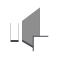“无关型”试题大展示

1已知多项式 x 2+ axy+ bbx 23 x+ 6 y3的差的值与字母 x无关，求代数式 3( a 22 abb 2)–( 4 a 2+ ab+ b 2)的值．

∵多项式 x 2+ axy+ bbx 23 x+ 6 y3的差的值与字母 x无关，

1b= 0a+ 3= 0

3( a 22 abb 2)–( 4 a 2+ ab+ b 2)=– a 27 ab4 b 2,

b= 1a=– 3，代入，得原式=–(– 3) 27×(– 314× 1 2= 8.

2如果关于 x的整式( 8 x 26 ax+ 14)–( 8 x 26 x+ 5)的值与 x无关，你知道 a应该取什么值吗？试试看

∵多项式( 8 x 26 ax+ 14)–( 8 x 26 x+ 5)的值与 x无关，

x的系数– 6 a+ 6= 0解得 a= 1.

3李华老师给学生出了一道题：当 x= 0.16y=– 0.2时，求 6 x 3 -2 x 3 y -4 x 3+ 2 x 3 y -2 x 3+ 15的值．题目出完后，小明说：“老师给的条件 x= 0.16y=– 0.2是多余的”．王光说：“不给这两个条件，就不能求出结果，所以不是多余的．”你认为他们谁说的有道理?为什么